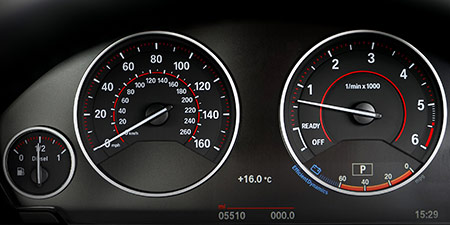# Miles per hour (mph) to Knot (kn)

Units of speed ﹣ Converter Miles per hour to Knot

Here you can convert the Speed unit Miles per hour into the unit Knot and vice versa you can convert Knot into Miles per hour. By clicking the "Swap units" icon, you will always obtain the desired conversion in the calculation result, i.e., mph to kn or kn to mph. With the following calculator you can also calculate any other Speed unit.

## Info about "Miles per hour"

The unit of length known as a "mile" and it should not be confused with the nautical mile and very commonly used in the Anglo-American countries. Therefore, the metric system is defined in miles (instead of kilometers) per time unit. For example, if a vehicle travels at a speed of 100 miles per hour (100 mph), it will move around 160 km per hour in the metric system.

The units are defined as follows: An object moving at 1 "mph" per hour will cover a distance of one mile. Therefore, 1 mile is equivalent to 1.609344 kilometers.

Definition: An object that moves with a knot for one hour will cover a distance of one nautical mile. Therefore 1 nautical mile is equivalent to 1,852 meters. A knot refers to the speed of one nautical mile per hour.

The following rule is an easy way to remember when converting knots into km/h: "Multiply by two, and minus 10%". For example, a ship travelling at 20 knots will have a speed around 40-4=36 km/h (exactly 37.04 km/h).

## Basis for conversion Miles per hour (mph) to Knot (kn) and vice versa

The abbreviation for the "Speed unit Miles per hour" is mph. The abbreviation for the "Speed unit Knot" is kn.

## Formula for the conversion of Miles per hour (mph) to Knot (kn) and vice versa

The calculation from Miles per hour to Knot shall be made using the following conversion formula:

Conversion formula Miles per hour to Knot

Determine the number of Knot from Miles per hour

Miles per hour × 0.86897624155103

## Formula for the conversion of Knot (kn) to Miles per hour (mph)

The calculation from Knot to Miles per hour shall be made using the following conversion formula:

Conversion formula Knot to Miles per hour

Determine the number of Miles per hour from Knot

Knot × 1.1507794484865

## Overview table : How many Miles per hour are in a Knot ?

Miles per hour mph ⇒ Knot kn
0.01 mph  are  0.00869 kn
0.02 mph  are  0.01738 kn
0.03 mph  are  0.02606 kn
0.04 mph  are  0.03475 kn
0.05 mph  are  0.04344 kn
0.06 mph  are  0.05213 kn
0.07 mph  are  0.06082 kn
0.08 mph  are  0.06951 kn
0.09 mph  are  0.07820 kn
0.10 mph  are  0.08689 kn
0.20 mph  are  0.17379 kn
0.30 mph  are  0.26069 kn
0.40 mph  are  0.34759 kn
0.50 mph  are  0.43448 kn
0.60 mph  are  0.52138 kn
0.70 mph  are  0.60828 kn
0.80 mph  are  0.69518 kn
0.90 mph  are  0.78207 kn
1 mph  corresponds to  0.86897 kn
2 mph  are  1.73795 kn
3 mph  are  2.60692 kn
4 mph  are  3.47590 kn
5 mph  are  4.34488 kn
6 mph  are  5.21385 kn
7 mph  are  6.08283 kn
8 mph  are  6.95181 kn
9 mph  are  7.82078 kn
10 mph  are  8.68976 kn
20 mph  are  17.37952 kn
30 mph  are  26.06928 kn
40 mph  are  34.75905 kn
50 mph  are  43.44881 kn
60 mph  are  52.13857 kn
70 mph  are  60.82833 kn
80 mph  are  69.51809 kn
90 mph  are  78.20786 kn
100 mph  are  86.89762 kn
200 mph  are  173.79524 kn
300 mph  are  260.69287 kn
400 mph  are  347.59049 kn
500 mph  are  434.48812 kn
600 mph  are  521.38574 kn
700 mph  are  608.28336 kn
800 mph  are  695.18099 kn
900 mph  are  782.07861 kn
1 000 mph  are  868.97624 kn

## Overview table : How many Knot are in a Miles per hour ?

Knot kn ⇒ Miles per hour mph
0.01 kn  are  0.01150 mph
0.02 kn  are  0.02301 mph
0.03 kn  are  0.03452 mph
0.04 kn  are  0.04603 mph
0.05 kn  are  0.05753 mph
0.06 kn  are  0.06904 mph
0.07 kn  are  0.08055 mph
0.08 kn  are  0.09206 mph
0.09 kn  are  0.10357 mph
0.10 kn  are  0.11507 mph
0.20 kn  are  0.23015 mph
0.30 kn  are  0.34523 mph
0.40 kn  are  0.46031 mph
0.50 kn  are  0.57539 mph
0.60 kn  are  0.69046 mph
0.70 kn  are  0.80554 mph
0.80 kn  are  0.92062 mph
0.90 kn  are  1.03570 mph
1 kn  corresponds to  1.15077 mph
2 kn  are  2.30155 mph
3 kn  are  3.45233 mph
4 kn  are  4.60311 mph
5 kn  are  5.75389 mph
6 kn  are  6.90467 mph
7 kn  are  8.05545 mph
8 kn  are  9.20623 mph
9 kn  are  10.35701 mph
10 kn  are  11.50779 mph
20 kn  are  23.01558 mph
30 kn  are  34.52338 mph
40 kn  are  46.03117 mph
50 kn  are  57.53897 mph
60 kn  are  69.04676 mph
70 kn  are  80.55456 mph
80 kn  are  92.06235 mph
90 kn  are  103.57015 mph
100 kn  are  115.07794 mph
200 kn  are  230.15589 mph
300 kn  are  345.23383 mph
400 kn  are  460.31177 mph
500 kn  are  575.38972 mph
600 kn  are  690.46766 mph
700 kn  are  805.54561 mph
800 kn  are  920.62355 mph
900 kn  are  1 035.70150 mph
1 000 kn  are  1 150.77944 mph